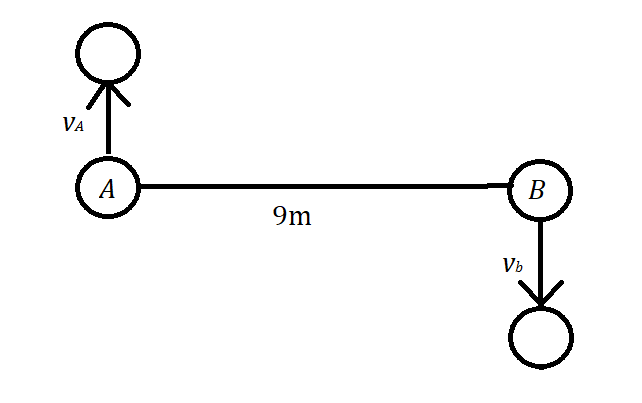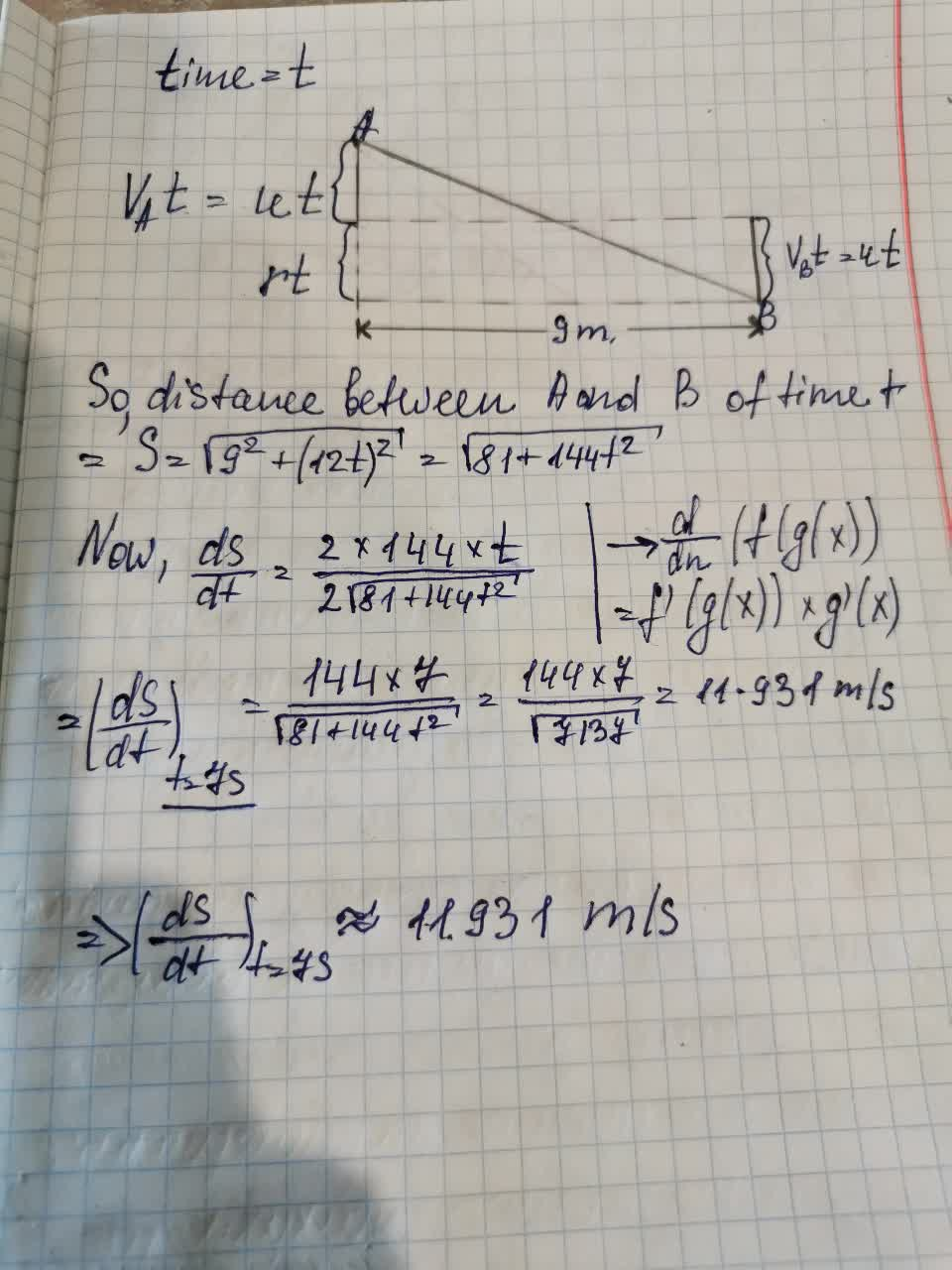# Ball A moved at a speed of vA=4 m/s and ball B moved at a speed of vb=8 m/s in the directions as shown in the figure below.Maiclubk 2021-09-12 Answered
Two balls A and B were Initially 9 m apart. Ball A moved at a speed of vA=4 m/s and ball B moved at a speed of vb=8 m/s in the directions as shown in the figure below.• Questions are typically answered in as fast as 30 minutes

### Plainmath recommends

• Get a detailed answer even on the hardest topics.
• Ask an expert for a step-by-step guidance to learn to do it yourself.Alix Ortiz

The answer is given below in the photo